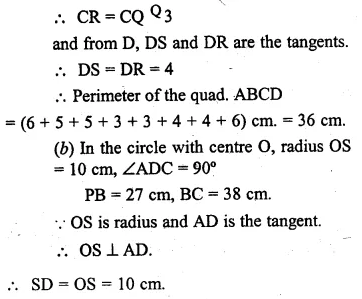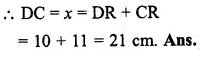Guru

# Question 7. (a) In figure (i) given below, quadrilateral ABCD is circumscribed; find the perimeter of quadrilateral ABCD. (b) In figure (ii) given below, quadrilateral ABCD is circumscribed and AD ⊥ DC ; find x if radius of incircle is 10 cm.

• 0

One of the most important and exam oriented question from Chapter name- circles
Topic – Angle properties of circles
Chapter number- 15

We have been given that quadrilateral  is circumscribed; we have find the perimeter of quadrilateral .

B) in figure (ii) given below, quadrilateral ABCD is circumscribed and AD ⊥ DC ; we have to find x if radius of incircle is 10 cm.
ICSE Avichal publication
Understanding ICSE Mathematics
Question 7

Share